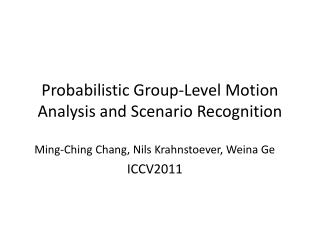DownloadDownload PresentationProbabilistic Group-Level Motion Analysis and Scenario Recognition

# Probabilistic Group-Level Motion Analysis and Scenario Recognition

Download Presentation## Probabilistic Group-Level Motion Analysis and Scenario Recognition

- - - - - - - - - - - - - - - - - - - - - - - - - - - E N D - - - - - - - - - - - - - - - - - - - - - - - - - - -
##### Presentation Transcript

1. Probabilistic Group-Level Motion Analysis and Scenario Recognition Ming-Ching Chang, Nils Krahnstoever, Weina Ge ICCV2011

2. Outline • Introduction • Related Works • Probabilistic Group Analysis • Probabilistic Group Structure Analysis and • Scenario RecognitionProbabilistic Individual Motion and Interaction Analysis • Experiment Results

3. Introduction • Environments such as schools, transportation hubs are typically characterized by a large number of people with complex social interactions. • Understanding group-level interaction is particularly important in surveillance and security.

4. Introduction • The gang are the root cause of criminal behavior. • A major goal of this work is to automatically detect and predict events of interest by understanding behaviors and activities at the group level.

5. Introduction • There are at least three major issues in performing group-level behavior recognition. • 1.define the groups • 2.relationships change rapidly • 3. efficient inference strategy

6. Introduction • This paper perform and maintain a soft grouping structure throughout the entire event recognition stage. • A key feature of our soft grouping strategy is that group-level activities must be represented on a per individual basis.

7. Introduction • based on two components: • A probabilistic group analysis • A probabilistic motion analysis • handling arbitrary number of individuals. • The group representation is general and can be combined naturally

8. Related Works • Ni et al.  recognize human group activities using three types of localized causalities based on training and labeling. • Ryoo and Aggarwal  simultaneously estimate group structure and detect group activities using a stochastic grammar. • For pedestrian tracking and motion prediction, most existing methods focus on modeling simple activities of a single person or theinteractivity between two.

9. Probabilistic Group Analysis • we define a pairwise grouping measure based on three main terms: the distance between two individuals, the motion (body pose and velocity) and the track history.

10. Probabilistic Group Analysis • For instantaneous affinity measure

11. Probabilistic Group Analysis • i and j are connected

12. Probabilistic Group Analysis • We then set the connection probability between i and j to be the optimal path amongst all possible paths, which yields the highest probability

13. Probabilistic Group Structure Analysis and Scenario Recognition • Group scenario recognition: • In security, group loitering is of particular interest to municipalities, because it is likely related to (or often the prologue of) illegal activities.

14. Probabilistic Group Structure Analysis and Scenario Recognition • definition of a loitering person has three criteria: • is currently moving slowly • has been close to the current position at a point in time at Tmin~Tmax ago • Was also moving slowly at that previous point in time

15. Probabilistic Group Structure Analysis and Scenario Recognition • Two groups of a pair of people i and j are considered currently close-by if there exists no person k that both i and j are close to. • The distinct groups between individuals i and j ,there is no connectivity from (i, k) or (k, j),

16. Probabilistic Individual Motion and Interaction Analysis • Contain three main categories: • individual motion types • relative motion direction between pairs • relative distance change between pairs

17. Probabilistic Individual Motion and Interaction Analysis

18. Probabilistic Individual Motion and Interaction Analysis

19. Probabilistic Individual Motion and Interaction Analysis • These can be combined to detect high-level scenarios. • For example, the probability of two targets quickly running toward, and meeting at the same location is:

20. Experiment Results

21. Experiment Results

22. Experiment Results

23. Experiment Results